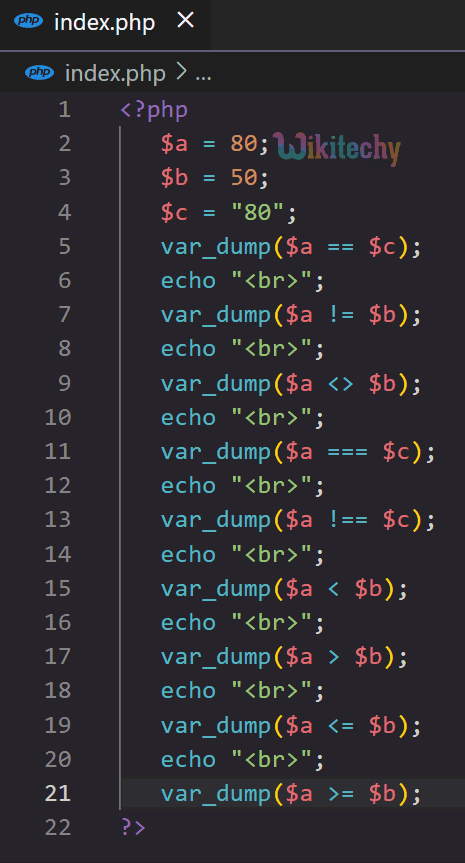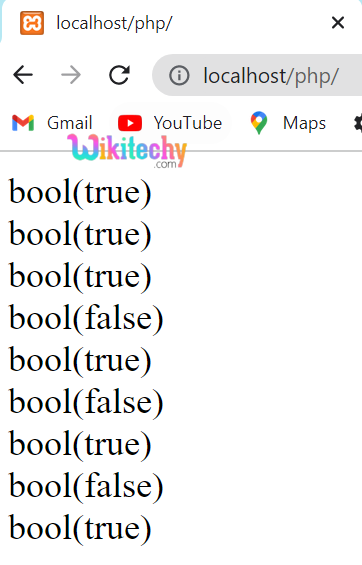# PHP Comparison Operators - Comparison Operators in PHP

## PHP Comparison Operators

• PHP comparison operators are used to compare two values (number or string).
• These operators are used in conditional statements (like if/else) to compare values and return a boolean value (true or false).
Operator Syntax Operation
Equal To (==) \$a == \$b Returns True if both the operands are equal
Not Equal To (!=) \$a != \$b Returns True if both the operands are not equal
Not Equal To (<>) \$a <> \$b Returns True if both the operands are unequal
Identical (===) \$a === \$b Returns True if both the operands are equal and are of the same type
Not Identical (!==) \$a == \$b Returns True if both the operands are unequal and are of different types
Less Than (<) \$a < \$b Returns True if \$a is less than \$b
Greater Than (>) \$a > \$b Returns True if \$a is greater than \$b
Less Than or Equal To (<=) \$a <= \$b Returns True if \$a is less than or equal to \$b
Greater Than or Equal To (>=) \$a >= \$b Returns True if \$a is greater than or equal to \$b## Output